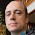## Thursday, March 14, 2019

### Adding support for embedding Latex equations in DITA content

LaTeX is a high-quality typesetting system that includes features designed for the production of technical and scientific documentation. LaTeX can also be used to express mathematical formulas in a textual format. By default, web browsers and PDF readers do not have support to show mathematical equations written in LaTeX but there are open source projects that can read LaTeX and convert it to other image types.

Adding support for writing LaTeX equations in DITA topics would imply three stages:
• Find a way to write the equation in the DITA XML content. You can either create a DITA DTD specialization and add a new element (for example, called <latex> and it extends the DITA <foreign> element). Alternatively, you can use the DITA <foreign> element with a specific @outputclass attribute value directly:
<!DOCTYPE topic PUBLIC "-//OASIS//DTD DITA Topic//EN" "topic.dtd">
<topic id="testEquation">
<title>Test equation</title>
<body>
<p><foreign outputclass="embed-latex">L' = {L}{\sqrt{1-\frac{v^2}{c^2}}}</foreign></p>
</body>
</topic>
• If you want Oxygen to properly present the LaTeX equation when editing in the Author visual mode, you need an Oxygen plugin that converts the equation content to an image. There is a sample plugin that does that here: https://github.com/oxygenxml/wsaccess-javascript-sample-plugins/tree/master/latex-images-support. You can download and copy the plugin folder latex-images-support to the Oxygen plugins folder, then restart Oxygen.
• Publishing to HTML-based and PDF outputs. This DITA Open Toolkit plugin automatically converts LaTeX images to SVG when publishing: https://github.com/oxygenxml/dita-latex.

1.1.